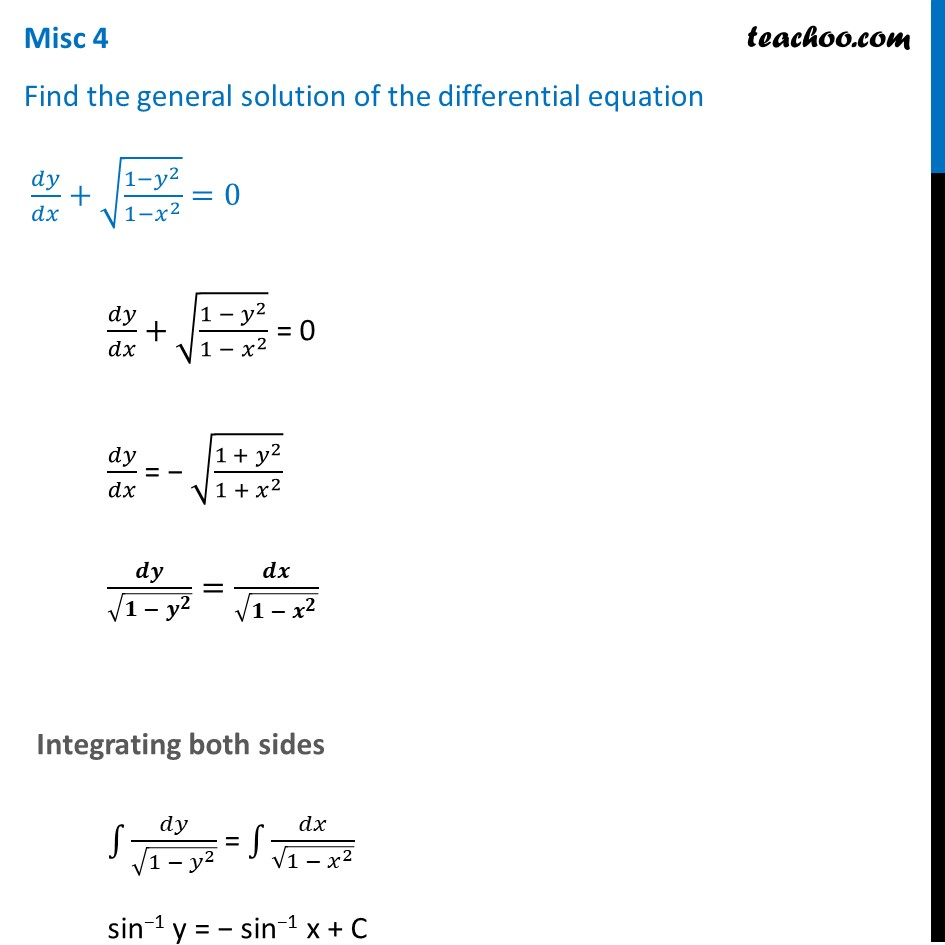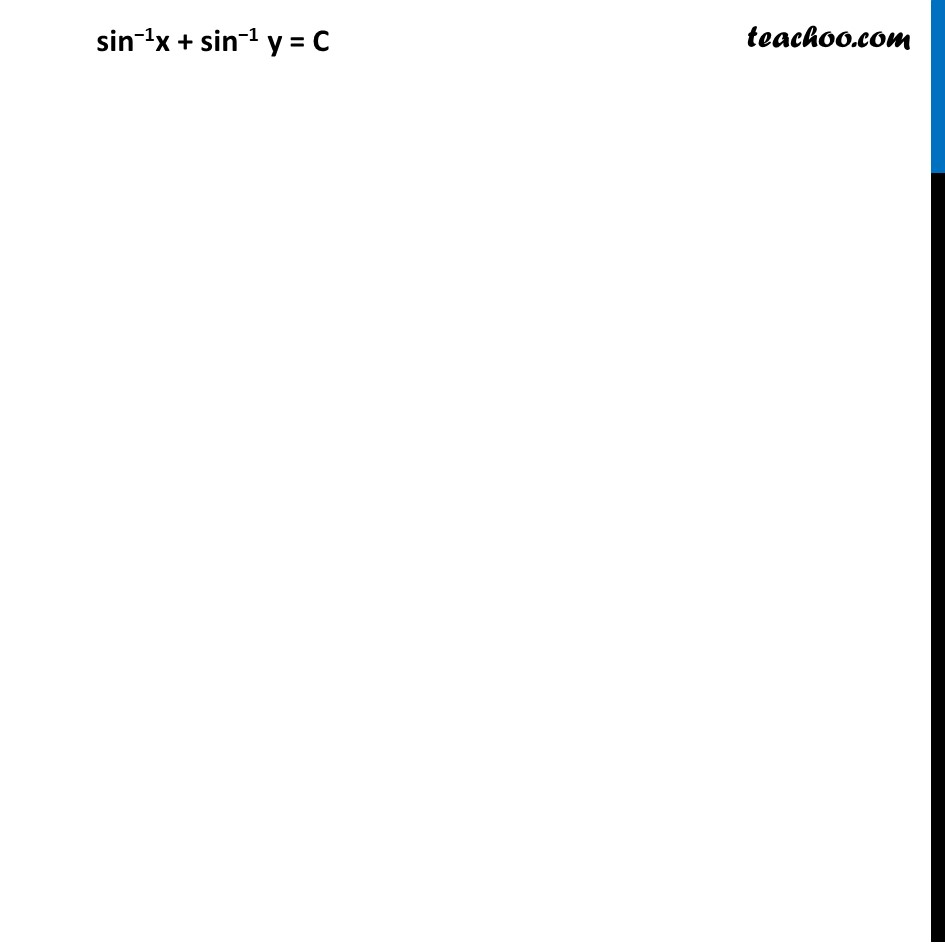Miscellaneous

Chapter 9 Class 12 Differential Equations
Serial order wiseLearn in your speed, with individual attention - Teachoo Maths 1-on-1 Class

### Transcript

Misc 4 Find the general solution of the differential equation 𝑑𝑦/𝑑𝑥+√((1−𝑦^2)/(1−𝑥^2 ))=0𝑑𝑦/𝑑𝑥+√((1 − 𝑦^2)/(1 − 𝑥^2 )) = 0 𝑑𝑦/𝑑𝑥 = − √((1 + 𝑦^2)/(1 + 𝑥^2 )) 𝒅𝒚/√(𝟏 − 𝒚^𝟐 )=𝒅𝒙/√(𝟏 − 𝒙^𝟐 ) Integrating both sides ∫1▒𝑑𝑦/√(1 − 𝑦^2 ) = ∫1▒𝑑𝑥/√(1 − 𝑥^2 ) sin−1 y = − sin−1 x + C sin−1x + sin−1 y = C